# Borsuk-Ulam theorem

(diff) ← Older revision | Latest revision (diff) | Newer revision → (diff)

In the Borsuk–Ulam theorem (K. Borsuk, 1933 [a2]), topological and symmetry properties are used for coincidence assertions for mappings defined on the-dimensional unit sphere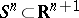. Obviously, the following three versions of this result are equivalent:

1) For every continuous mapping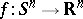, there exists anwith.

2) For every odd continuous mapping, there exists anwith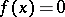.

3) If there exists an odd continuous mapping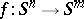, then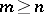. The Borsuk–Ulam theorem is equivalent, among others, to the fact that odd continuous mappingsare essential (cf. Antipodes), to the Lyusternik–Shnirel'man–Borsuk covering theorem and to the Krein–Krasnosel'skii–Mil'man theorem on the existence of vectors "orthogonal" to a given linear subspace [a3].

The Borsuk–Ulam theorem remains true:

a) if one replacesby the boundary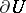of a bounded neighbourhoodof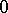with;

b) for continuous mappings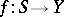, whereis the unit sphere in a Banach space,,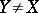, a linear subspace ofand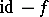a compact mapping (for versions 1) and 2)).

For more general symmetries, the following extension of version 3) holds:

Letandbe finite-dimensional orthogonal representations of a compact Lie group, such that for some prime number, some subgroup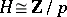acts freely on the unit sphere. If there exists a-mapping, then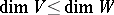.

For related results under weaker conditions, cf. [a1]; for applications, cf. [a4].

How to Cite This Entry:
Borsuk-Ulam theorem. Encyclopedia of Mathematics. URL: http://encyclopediaofmath.org/index.php?title=Borsuk-Ulam_theorem&oldid=16557
This article was adapted from an original article by H. Steinlein (originator), which appeared in Encyclopedia of Mathematics - ISBN 1402006098. See original article